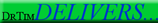go back (07.06.05/02.21.09)DrTim homepage link

Intro to Clinical Statistics
by Timothy Bilash MD
July 2005

based on:
Review of Basic & Clinical Biostatistics
Beth Dawson, Robert Trapp (2001)

From CH6 and Ch10

I. TWO SEPARATE OR INDEPENDENT GROUPS (from Chapter 6)

1. Levine Test (p141)

1. Levine Test is an alternative test for the equality of means

1. Utilizes absolute deviations from the mean
2. Tests the hypothesis that the average of the absolute value of the observation deviations from the mean is the same in each group.
3. Two-Sample T-Test is done, using the absolute value of the deviations (absolute distance each observation is from the mean in that group) rather than the deviations
4. If the T Test on these deviations is significant (>.05), the hypothesis of equal variance is rejected and the T-Test on the difference of means is not appropriate.
1. if the Levine Test is significant, the deviations from the mean in one group on average exceeds the other
5. valid for normal and nonnormal population distributions
6. a modified Levine Test replaces the mean with median

2. [ANOTE] Statistical Significance [[ ]] ?expected value meaning

1. statistical significance is determined by the deviations of the observed values from some expected value, not the observed values themselves. whether a test is done for equality of the expected value, or equality for some function of the expected value, want the deviations from the expected to be minimized.
1. deviation = y-f(y)
1. mean: f(y)=ybar
2. least squares: f(y)=a+bx
2. identical population distributions may be more important than equal population variances for validity of the T-Test.

2. Welch Test (p141)
1. another test for comparing means in two independent groups

• II. STATISTICAL METHODS FOR MULTIPLE VARIABLES (from Chapter 10) [[ ]]

1. Predicting Group Outcomes (Nominal <-> Categorical <-> Grouped)

1. Three methods are used for Outcomes measured on nominal scale (bi-valued: present or not-present, yes or no, true or false, + or - ) [[ ]]

1. Logistic Regression (Curve Fitting)
1. can be transformed into Odds Ratio
2. Controls (adjusts) for confounding variables using analysis of co-variance
2. Discriminant Analysis
1. used less now
3. Log-linear Analysis
1. rarely used

2. Logistic Regression (Curve Fitting) [[ ]]

1. Fits an exponential (log-linearized) to the data (log-linearized), obtaining a regression coefficient (bi) for each factor

1. Probability of outcome is divided up among the (N in number) X factors
= 1 / [1 + exp(b0 + b1 X1 + b2X 2 + ... + bNXN )] = CONSTANT
2. Independent Variables are then selected or derived independently from the fit [[ ]]???

2. Multiple outcomes vs multiple risks

1. Logistic Regression - find best fit for multiple Risk Factors
1. Multiple Risk Factors are Independent variables that are Numerical or Nominal(Categories) , ie, X's
2. Single Outcome Variable is bi-valued (Logical), ie, Y, or can also be used for Multiple Outcomes if Categories
3. Sometimes also called multiple regression

2. Multiple Regression - find best fit for multiple Outcome Data, holding the values of all other variables constant
1. Sometimes used to mean combination of both Multiple Risk Factors with Multiple Outcome Data (note confusion between these different uses)

3. If the independent variables (predictors or risk factors) are bi-valued, then can interpret regression coefficients as Odds Ratio

1. odds ratio is a summary statistic
2. odds ratio is the outcome probability ratio for a bi-valued risk
3. contrast a 95% chance in one patient with 100% chance in 95% of patients
1. equivalent if odds ratio is constant
2. an underlying problem for survival curves to distinguish between these (especially if time affects outcomes or risks)

4. Chi-square Test is used to determine significance for each variable's regression coefficient when the Outcome is Multiple Categories/Nominal (cant use a T or F test)

5. T or F test can be used if single input and single output variables to determine whether each regression coefficient is different from zero (if one binary risk and one binary outcome - see elsewhere)

6. T Distribution can be used to form confidence intervals for each regression coefficient

1. If 95% confidence interval for the odds ratio does not include value of one, then 95% confident that the factor associated with the odds ratio is a significant factor within the confidence interval

7. Regression tends to underpredict the probability that a risk factor is present for a given outcome
1. some advocate the Kappa Statistic for more correct percentage (but see R Wilcox for disagreement with this)page views since Sept2007